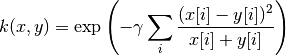# 5.8. 成对的矩阵, 类别和核函数

The sklearn.metrics.pairwise 子模块实现了用于评估成对距离或样本集合之间的联系的实用程序。

1. 对于所有的 a 和 b，d(a, b) >= 0
2. 正定性：当且仅当 a = b时，d(a, b) == 0
3. 对称性：d(a, b) == d(b, a)
4. 三角不等式：d(a, c) <= d(a, b) + d(b, c)

1. S = np.exp(-D * gamma), 其中 gamma 的一种选择是 1 / num_features
2. S = 1. / (D / np.max(D))

X 的行向量和 Y 的行向量之间的距离可以用函数 pairwise_distances 进行计算。 如果 Y 被忽略，则 X 的所有行向量的成对距离就会被计算。 类似的，函数 pairwise.pairwise_kernels 可以使用不同的核函数(kernel functions)来计算 X 和 Y 之间的 kernel。 请查看API获得更多详情。

>>> import numpy as np
>>> from sklearn.metrics import pairwise_distances
>>> from sklearn.metrics.pairwise import pairwise_kernels
>>> X = np.array([[2, 3], [3, 5], [5, 8]])
>>> Y = np.array([[1, 0], [2, 1]])
>>> pairwise_distances(X, Y, metric='manhattan')
array([[ 4.,  2.],
[ 7.,  5.],
[12., 10.]])
>>> pairwise_distances(X, metric='manhattan')
array([[0., 3., 8.],
[3., 0., 5.],
[8., 5., 0.]])
>>> pairwise_kernels(X, Y, metric='linear')
array([[ 2.,  7.],
[ 3., 11.],
[ 5., 18.]])


## 5.8.1. 余弦相似度

cosine_similarity 计算L2正则化的向量的点积. 也就是说, if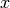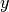都是行向量,, 它们的余弦相似度定义为: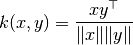## 5.8.2. 线性核函数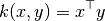## 5.8.3. 多项式核函数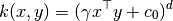## 5.8.4. Sigmoid 核函数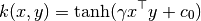## 5.8.5. RBF 核函数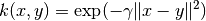## 5.8.6. 拉普拉斯核函数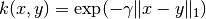## 5.8.7. 卡方核函数

>>> from sklearn.svm import SVC
>>> from sklearn.metrics.pairwise import chi2_kernel
>>> X = [[0, 1], [1, 0], [.2, .8], [.7, .3]]
>>> y = [0, 1, 0, 1]
>>> K = chi2_kernel(X, gamma=.5)
>>> K
array([[1.        , 0.36787944, 0.89483932, 0.58364548],
[0.36787944, 1.        , 0.51341712, 0.83822343],
[0.89483932, 0.51341712, 1.        , 0.7768366 ],
[0.58364548, 0.83822343, 0.7768366 , 1.        ]])

>>> svm = SVC(kernel='precomputed').fit(K, y)
>>> svm.predict(K)
array([0, 1, 0, 1])



>>> svm = SVC(kernel=chi2_kernel).fit(X, y)
>>> svm.predict(X)
array([0, 1, 0, 1])